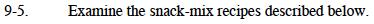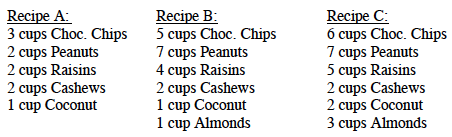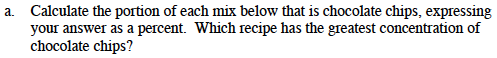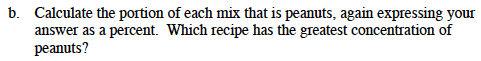### Home > MC1 > Chapter 9 > Lesson 9.1.1 > Problem9-5

9-5.For Recipe A, find the total number of cups in the recipe.

Next, write a fraction expressing the portion that is chocolate chips.

Convert the fraction into a percent.

3 cups + 2 cups + 2 cups + 2 cups + 1 cup = 10 cups

$\frac{3}{10}$

$\frac{3}{10}=\frac{30}{100}=30\%$

30% of Recipe A is chocolate chips.
Repeat the above steps for Recipe B and Recipe C, then determine which has the highest percent of chocolate chips.This problem is very similar to part (a), and you have already found the total number of cups in each recipe.
For each recipe, express the portion first as a fraction and then convert the fraction to a percent.